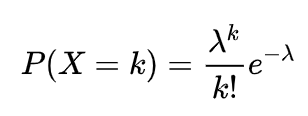生活中de泊松分布1) 将每次T之内观察的事件E发生的数量记录 (平均每天客人数量, 则T=一天)

2) 多个T的平均值找到 (比如过去几天, 平均每天来了2.1人), E(T) = λ = n*p = 2.1(用无限大的n来切分T至很小的一个时空区间 t, 保证区间之间的时间独立性; p则为该t之内的事件概率); 所以λ已知, 也就是发生事件的平均次数是知道的.

3) T时间来k个客人的概率是 P(x=k) = lim (n,k) p^k * (1-p)^(n-k) = (当n无穷大的时候) e^(-λ) * λ^k / k!

4) 这样可以把概率分布柱状图给画出来 P(x=1), P(x=2), …

Contents